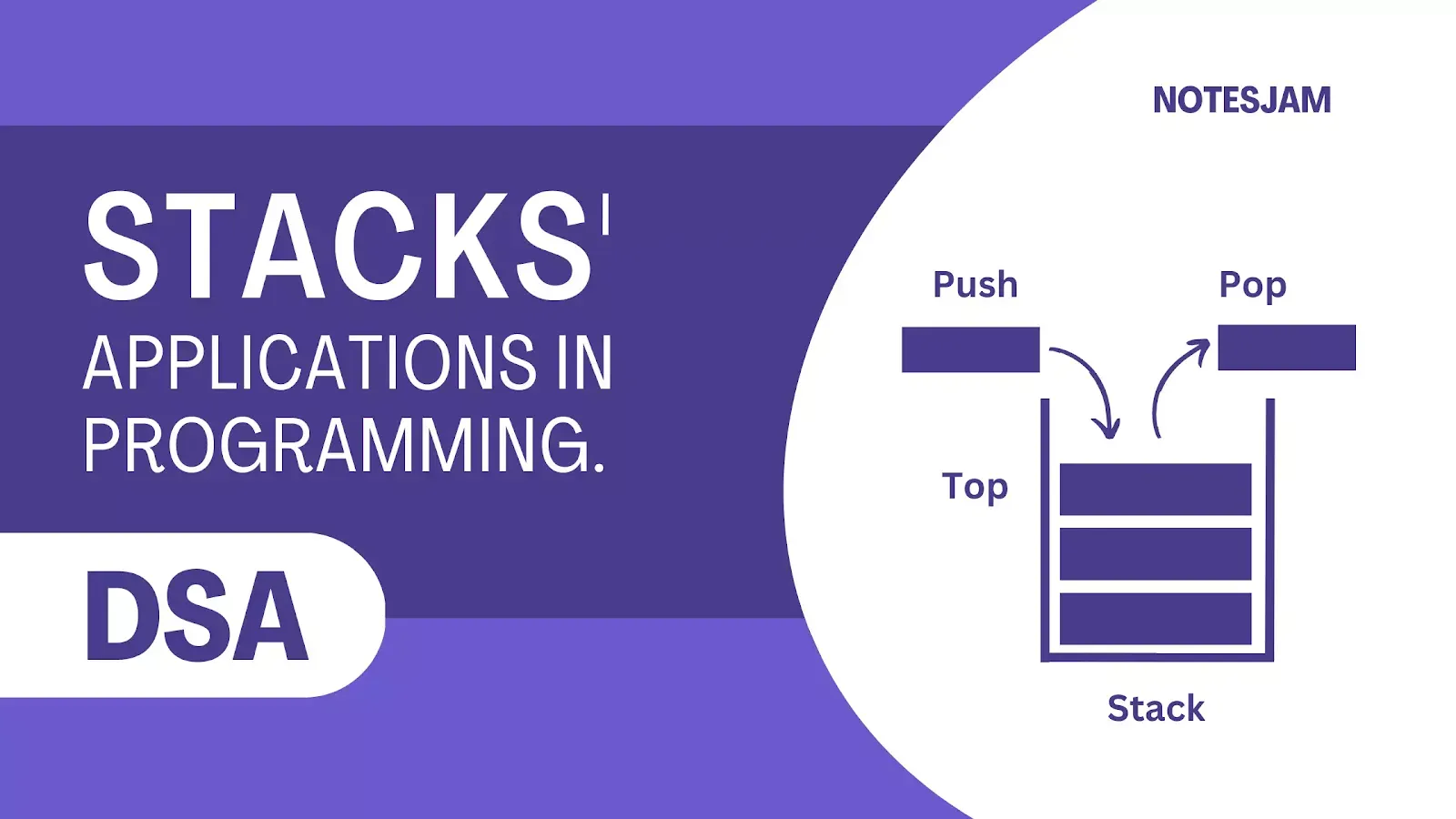# Introduction to Stacks and Their Applications in ProgrammingSave important notes and articles from internet using arusaquotes app.

In computer science, a stack is a data structure that allows data to be added and removed in a particular order. A stack follows the Last-In-First-Out (LIFO) principle, meaning the last item added to the stack is the first item to be removed.

The stack data structure is commonly used in programming for many reasons, including its simplicity, efficiency, and versatility.

In this article, we will explore the fundamentals of stacks, their applications in programming, and their implementation in various programming languages.

## Stack Operations

Stacks can be thought of as a collection of elements with two main operations: push and pop. The push operation adds an element to the top of the stack, and the pop operation removes an element from the top of the stack.

The push and pop operations are the only ways to add and remove elements from a stack, respectively.

In addition to push and pop, there are several other stack operations that are often used in programming, including:

• Peek: Returns the top element of the stack without removing it.
• isEmpty: Returns true if the stack is empty, false otherwise.
• isFull: Returns true if the stack is full (in case of a fixed-size stack), false otherwise.

## Applications of Stacks in Programming

Expression Evaluation: Stacks are commonly used in programming to evaluate arithmetic expressions. In this application, the operands and operators of an expression are added to the stack one by one.

When an operator is encountered, the top two operands are popped from the stack, the operation is performed, and the result is pushed back onto the stack. This process continues until the entire expression is evaluated.

Function Call Stack: When a function is called in a programming language, the return address and arguments are pushed onto the call stack. When the function returns, the return address is popped from the stack, and the program continues execution from the point where the function was called.

Undo/Redo Operations: Stacks are often used to implement undo and redo operations in software applications. Each time a user performs an action, the state of the application is saved on the stack.

If the user wants to undo the last action, the state is popped from the stack, and the application reverts to the previous state. If the user wants to redo the last action, the state is pushed back onto the stack, and the application returns to the current state.

Backtracking: Stacks are used in backtracking algorithms to store the current state of the search. When a dead end is reached, the state is popped from the stack, and the search continues from the previous state.

## Stack Implementation in Programming Languages

Stacks can be implemented in various programming languages using arrays, linked lists, or dynamic arrays. Arrays are the simplest implementation of a stack, where the stack elements are stored in a contiguous block of memory.

However, arrays have a fixed size, so their use is limited in dynamic applications. Linked lists, on the other hand, allow for a dynamic stack size, but require more memory and overhead due to the need to store pointers.

In Python, stacks can be implemented using lists. Lists in Python are dynamic arrays, which allow for the creation of stacks with a flexible size.

The append method can be used to add elements to the top of the stack, and the pop method can be used to remove elements from the top of the stack. Here is an example implementation of a stack in Python using a list:

``````stack = []

# Push operation
stack.append(1)
stack.append(2)
stack.append(3)

# Pop operation
top = stack.pop()
print(top) # Output: 3```
```

In C++, stacks can be implemented using arrays or linked lists. The array-based implementation requires a fixed-size array and a variable to keep track of the top of the stack.

The push operation increases the value of the top variable and adds the element to the array at the new top position. The pop operation retrieves the element at the top position, decreases the value of the top variable, and returns the element.

Here is an example implementation of a stack in C++ using an array:

``````#define MAX_SIZE 100

int stack[MAX_SIZE];
int top = -1;

// Push operation
void push(int element) {
if (top >= MAX_SIZE - 1) {
// Stack is full
return;
}
top++;
stack[top] = element;
}

// Pop operation
int pop() {
if (top < 0) {
// Stack is empty
return -1;
}
int element = stack[top];
top--;
return element;
}
```
```

In Java, stacks can be implemented using the Stack class provided in the Java Collections Framework. The Stack class is a subclass of the Vector class and provides methods for push, pop, peek, and isEmpty operations.

Here is an example implementation of a stack in Java using the Stack class:

``````import java.util.Stack;

Stack<Integer> stack = new Stack<Integer>();

// Push operation
stack.push(1);
stack.push(2);
stack.push(3);

// Pop operation
int top = stack.pop();
System.out.println(top); // Output: 3
```
```

## Conclusion

In summary, stacks are a fundamental data structure in computer science and have numerous applications in programming. They are simple, efficient, and versatile, making them a popular choice for many programming tasks.

Stacks can be implemented using arrays, linked lists, or dynamic arrays in various programming languages, including Python, C++, and Java.

Understanding stacks and their applications can help programmers write more efficient and effective code.